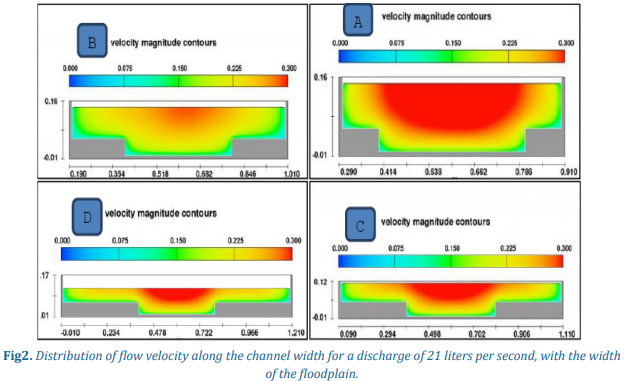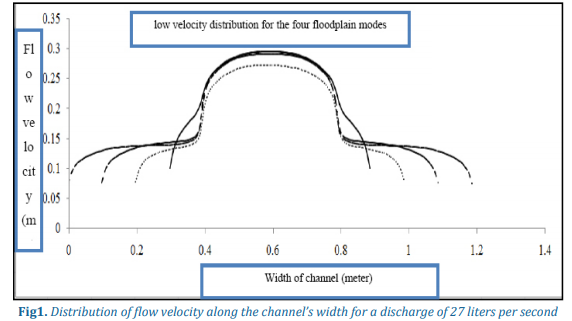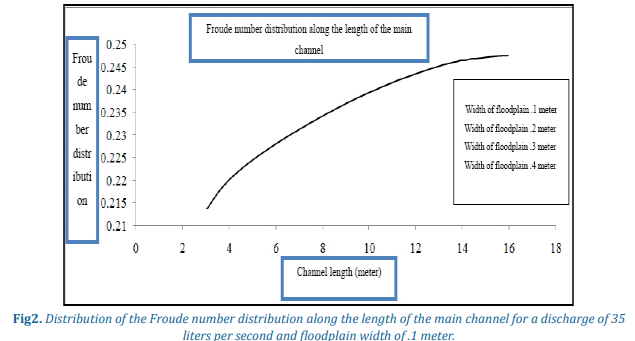## Numerical Analysis of Hydraulic Flow Characteristics in Prismatic Compound Channels Using Flow3D Software

Citation: Numerical Analysis of Hydraulic Flow Characteristics in Prismatic Compound Channels Using Flow3D Software, American Research Journal of Civil and Structural Engineering, vol 3, no. 1, pp. 1-10.

Copyright This is an open access article distributed under the Creative Commons Attribution License, which permits unrestricted use, distribution, and reproduction in any medium, provided the original work is properly cited

## Abstract:

Compound channels are hydraulic sections that consist of two parts including the main channel and the floodplain. The main channel conveys the usual runoffs and the basic discharge that often flows in the river. Predicting the flow characteristics in the prismatic compound channels includes the effect of the interaction between a fast flow in the main channel and a slower one in the floodplains. This speed difference creates a shear layer at the interface between the main channel and the floodplain, which results in the exchange of the size of the movement between the main channel and the floodplains. The result is a reduction in the flow-conveyance capacity of the main channel and its increase in the floodplains, which should be considered in flow modeling. On the other hand, Flow3D software is a great software for Computational Fluid Dynamics that is used to solve complex geometry problems. The main objective of this study is to evaluate the Flow3D model in numerical simulation of behavior of hydraulic flow characteristics such as velocity distribution, shear stress distribution and in prismatic compound channels. In this study, Volume of Fluid Method (VOF) was used to simulate water surface profiles and the dimensional Buckingham π theorem was used to extract parameters without effective dimension; besides, the model’s precision was tested by an experimental analysis in a rectangular flume. The results showed that the Flow3D numerical model has high accuracy in computing the flow passing the prismatic compound channel and has been able to model the hydraulic parameters of the flow and provide appropriate results.

Keywords: Prismatic Compound Channel, Floodplain, Numerical Modeling, Flow3D

## Description:

INTRODUCTION

An important aspect of rivers’ hydraulics is the lateral distribution of velocity and boundary shear stress. The rivers appear to be in the form of a compound cross section at their extreme ends, such that water overflows from the main channel of the river and enters the floodplains during floods. Due to their proximity to the river bank and their high latitude and fertile soil, floodplains have always been considered for various recreational, commercial, agricultural and residential purposes. Therefore, the awareness of the flow hydraulic in the floodplains is essential for the protection of humans, as well as the structures and installations in them.

The existence of floodplains in compound channels causes the flow to face an extensive wet surrounding as soon as it exits the main channel, resulting in imposing a considerable shear force to the flow due to the considerable exchange of mass and the movement size between the sub-sections of the channel.This shear force significantly reduces the flow velocity in the floodplainscompared to the main channel, and this velocity difference between the floodplain and the main channel leads to the formation of an interaction zonewhich produces a complicated flow in the compound channel that is seen as awhirlpool flow on the plan. Therefore, numerical simulation of the flow pattern of these channels is very complicated. Many researchers have worked on compound sections. The results of these studies have shown that the flow hydraulic in the compound sections is fundamentally different with simple sections. In compound sections, significant shear stress is created on the interface between the main section and the floodplains, which reduces the total flow discharge. This phenomenon was first described by Sellin (1964) and was also studied by researchers such as Myers and Elsawy (1975) and Werramliton et al. (1982). By conducting an experimental analysis on prismatic compound sections, Sellin (1964) showed that shear layer and strong structures of turbulence caused by the transfer of momentum from the main channel to the floodplains led to a decrease in the flow discharge of the entire section.Knight et al. (1984) presented an experimental method for calculating the average shear stress in the bed and wall of direct rectangular channels. Knight and Hamed(1984) carried out experiments to examine the distribution of apparent shear stress and presented equations for calculating the apparent shear force in compound prismatic sections with coarsefloodplains.Shiono and Knight (1998) presented a two-dimensional analytical model based on the Navier-Stokes equation for solving the lateral distribution of velocity and shear stress in simple and compound sections; finally, the effect of secondary flows was also considered by investigating the turbulent flow in the open channel with varying depth throughout the channel in 1990 and by modifying this model in1991. Using experimental data and based on Navier-Stokes equation, Ervine et al. (2000) developed two-dimensional analytic models for expressing the lateral distribution of velocity in prismatic compound channels. Moreover, Osman and Valentin (2006) used nonlinear K-ɛ turbulence model and Reynolds Stress Model to model velocity distribution in compound sections. Most of these studies focused on the calculation of flow discharge, apparent shear stress, distribution of depth averaged velocity and boundary shear stress in compound sections whileassuming uniform flow. Safarzadeh and Rezaei(2016) tried to conduct numerical simulation of the flow field in prismatic compound channels, including lateraldistribution of depth averaged velocity and shear stress by using the -ANSYS CFX software. Comparing the numerical simulation’s results obtained from solving theReynolds averaged Navier-Stokes equationusing K-ε turbulence model along with the experimental data show that there is a comparative interaction between them.

Numerical studies with Flow3D software are very limited in terms of flow passing a compound channel. In this regard, a study conducted by Najafian et al. (2016) can be referred to. They came up with physical and numerical hydraulic modeling of the flow through the compound sections of the non-prismatic and coarse floodplains. In this research, numerical modeling was performed using the Flow3D model. The results of the study showed that coarseness of the floodplains has a significant effect on flow characteristics. Comparing numerical results with the experimental model showed that the Flow3D model with the use of the RNGK-ε turbulence model has a good accuracy in simulating the flow in these sections.

A review of the studies showed that a small number of numerical modeling has been performed on the prismatic compound channel. Despite the development of computational and computerized systems as well as the existence of unmeasurable complexities in this experimental model, using numerical simulation with Flow3D software can be very effective in the hydrological investigation of such flows. Considering the importance of the issue, the hydraulic flow in the prismatic compound channel is simulated in the present study by using this software and its results are compared with the results of the experimental study.

MATERIALS AND METHODS

Efforts are made to first run and calibrate the Flow3D mathematical model for the prismatic compound channel using the experimental data Rezaii (2006). Then, the ability of Flow3D software to perform the numerical simulation of the flow pattern in the prismatic compound channel is evaluated. Experiments were carried out in a channel of 18 meters in length, ½ m in width and 1 meter in height, in which a prismatic compound channel is embedded so that the main channel’s width is 0.4 meters. Figure (1) shows the layout and characteristics of the used experimental channel Shayannejad et. al. (2017).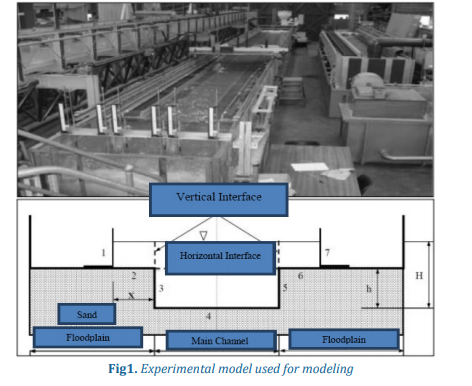The experimental results of the flow with different hydraulic flow and geometric conditions of the physical model were used to evaluate the numerical modeling of the flow in the prismatic compound channel (Table 1).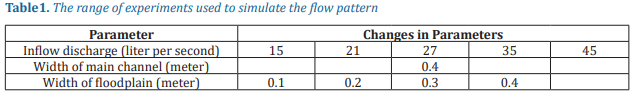DIMENSIONAL ANALYSIS

The first step in simulation and modeling is to identify the variables that affect the physical phenomenon. Dimensional analysis is a method in which the variables affecting the physical phenomenon are expressed in terms of dimensionless variables by using the concept of homogeneity of dimensions. Then, empirical relations are obtained based on these variables and experimental studies. To do dimensional analysis, there are several methodsincluding cataloging method, Buckingham π theorem, step by step method, and Hunziker and Wright Meyer method. In this study, the Buckingham π method, which has a wider application, is discussed and used.

The variables affecting the flow through the prismatic compound channel are:

1. Channel’s inflow discharge, Q; 2. Main channel width, B; 3. Floodplain width, b; 4. Channel length, L; 5. Main Channel’s Depth, H; 6. Depth of floodplain,hs ; 7. Depth of inflow, H; 8. Channeloutflow depth, Hd ; 9. Acceleration of gravity, g; 10. Specific weight of water, ρ; 11. Dynamic water viscosity, μ; (equation 1).

(1)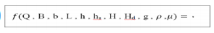Flow3D Software

Flow3D software is a powerful software based on limited volume method which has succeeded in numerical coding. Flow3D is a great software for fluid mechanics which is produced, developed, and supported by Flow Science, Inc.

Flow3D is a perfect model for solving complex fluid dynamics problems which is, compared to other models, user friendly and has a very powerful graphical interface that makes it easier to work with. This software uses a finite volume method to solve equations governing theflow by using regular networking and volume of fluid method to calculate free water level in open channels. Navier-Stokes equations are the basic equations used in this model; in this software, standard flow equations such as Navier-Stokes equations and continuity equations for the entire computing space are numerically solved.

Also, five turbulence models of Prandtl mixing length, one equation turbulence energy model, two equation k-ɛ model, renormalized group model, and large eddy simulation (LES) model are used to solve characteristics of turbulent flows.

This model includes many physical patterns including shallow waters, viscosity, cavitation, turbulence, and porous environments. The Flow3D model has a wide range of applications and capabilities in comparison with other models in the field of computational fluid dynamics.

In this software, standard flow equations such as Navier-Stokes equations and continuity equations for the entire computing space are numerically solved. The general form of the continuity equation is expressed as follows:

(2)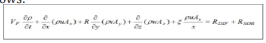Where VF is the coefficient of free flow towards flow and the value of R in the above equation is the coefficient of the coordinate in the form of Cartesian or cylindrical. The first expression on the right side of the continuity equation is related to the propagation of turbulence and can be defined as follows:

(3)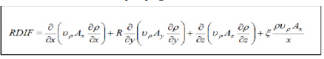The second expression on the right side of equation (3) denotes the origin of density, which is important for modeling the material mass injection.

(4)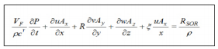The general form of momentum equations in the three-dimensional mode is as follows:

(5)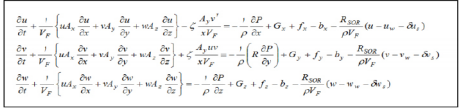In the above equations, Gx, Gy, and Gz are related to the volume acceleration. The parameters fx, fy, and fz are accelerations caused by viscous flows, and bx, by, and bz also contain equations related to decrease in porous environments.

SPECIFICATIONS OF THE SOLUTION FIELD

The boundary conditions used in the model and its range are such that the upstream boundary is selected based on Volume Flow Rate, the downstream boundary based on outflow, boundary in the bed based on wall conditions, and the boundary of the water surface is selected based on symmetry conditions (Table 2).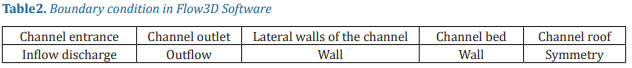To properly solve the governing equations, proper networking is one of the most important points that must be observed in numerical simulations. In all numerical experiments, the network dimensions were determined in a way that the network control parameters such as the network’sMaximum Aspect Ratio were selected along the longitudinal and depth directions and theMaximum Adjacent Cell Size Ratio were selected in different directions and in the vicinity of each other. In order to obtain accurate results, the values of each of the two parameters above should be close to 1, the network’s Maximum Aspect Ratio should be adjacent to each other, and the network’s Aspect Ratio in different directions should be1.25 and 3, respectively (Flossines, 2008 , OstadAli-Askari et. al. , 2015). Accordingly, in simulating the flow pattern, flow network meshing was considered as three-dimensional and the network dimensions in all the three dimensions were considered to be between 0/7 and 1 cm. The total number of meshes for each modeling is approximately 1,000,000 cells (Table 3).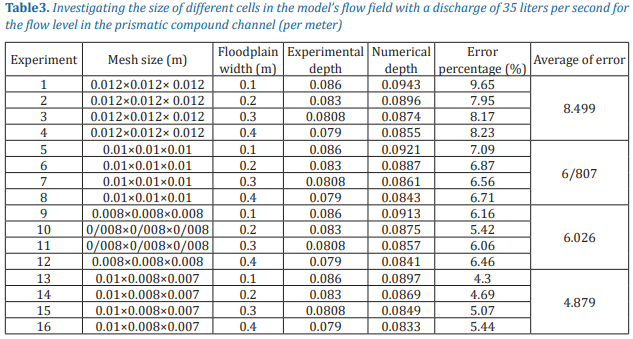In numerical modeling of hydraulic phenomena, one of the most important parameters used in calibration is the choice of the best turbulence model in order to more accurately simulate the hydraulic phenomenon. In this study, RNG k-ε, k-ε and LES turbulence models were evaluated in order to calibrate the model and simulate the flow (Shayannejad et al., 2015).

RESULTS AND DISCUSSION

In Fig. 2, the flow velocity distribution for the four floodplain modes is shown as color contours. Also, Chart (1) shows the effect of the floodplain width on the distribution of flow velocity across the channel. The results show that the maximum flow velocity for the floodplain width is 0.2 m below the rest of other states (Ostad-Ali-Askari et al., 2015).